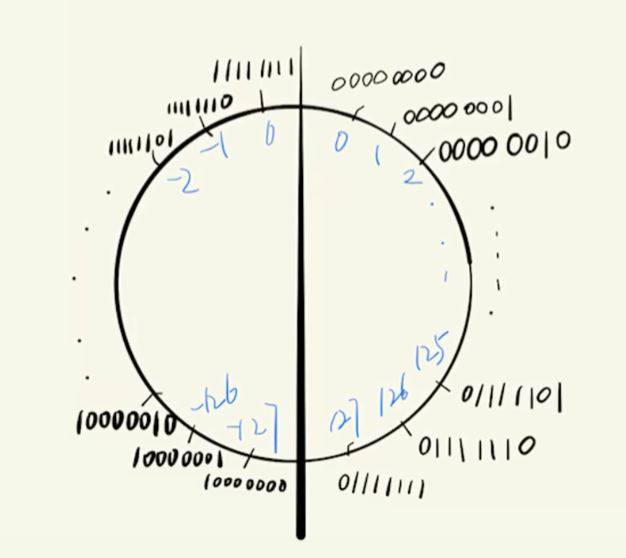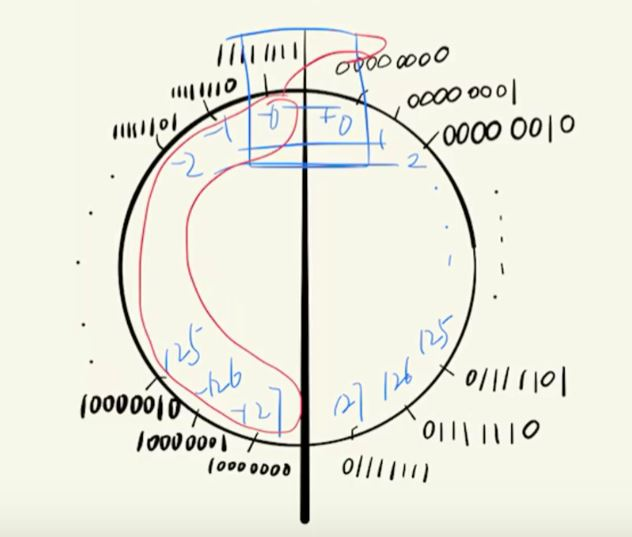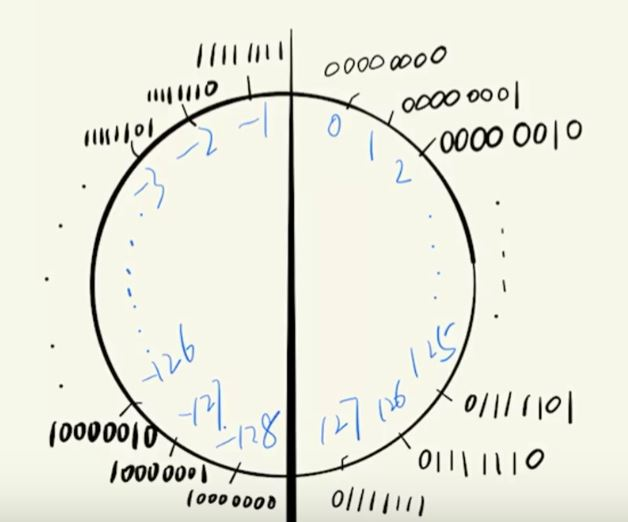补码

1. 用二进制表示正数或负数

$(2)_{10} = (010)_{2} , (-2)_{10}=(110)_2$

2. 用二进制数表示正数+负数

$(2)_{10} + (-2)_{10} = (00000010)_{2} + (11111101)_2 = (00000000)_2$（舍去最后进位的最高位）

3. 同时将一个十进制数只对应一个二进制编码4. 补码的计算方法

• $x\geq 0$那么，其补码就是$x$.
• $x < 0$ 那么，其补码就是$\overline{x} + 1$.
posted @ 2021-08-13 14:34  Maystern  阅读(108)  评论(0编辑  收藏  举报